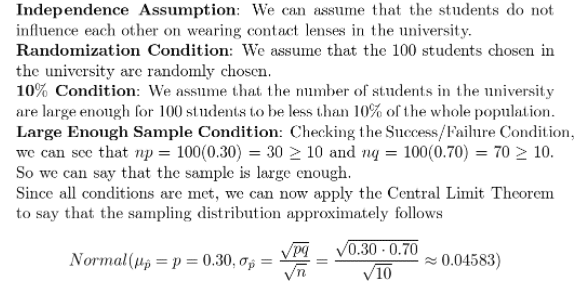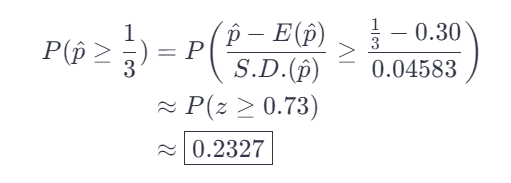# Assume That 30 Of Students At A University Wear Contact Lenses

We thoroughly check each answer to a question to provide you with the most correct answers. Found a mistake? Let us know about it through the REPORT button at the bottom of the page.

Assume that 30% of students at a university wear contact lenses.

a) We randomly pick 100 students. Let

p^​

represent the proportion of students in this sample who wear contacts. What’s the appropriate model for the distribution of

p^​?

Specify the name of the distribution, the mean, and the standard deviation. Be sure to verify that the conditions are met.

b) What’s the approximate probability that more than one-third of this sample wears contacts?

A) To check if we can apply the Central Limit Theorem in a particular experiment, we have to check the following conditions: Independence assumption, randomization condition, 10% condition, and Large Enough sample condition.

Given that p = 0.30 is the percentage of students in the university that has contact lenses and n = 100 which is the number of samples we got, the experiment is checked using these conditions:B) In getting the probability of the getting more than 1/3 who wears contact tenses in the sample, we use the normal distribution stated at (16a) and standardize it, such that,